NEET  >  The Gas Laws

# The Gas Laws - Notes | Study Chemistry Class 11 - NEET

 Table of contentsBoyle's LawCharles' LawPressure-Temperature Law (Gay Lussac's Law)Avogadro's Law1 Crore+ students have signed up on EduRev. Have you?

Boyle's Law

It relates the volume and the pressure of a given mass of a gas at constant temperature.

Boyle's law states that, at constant temperature, the volume of a sample of a gas varies inversely with the pressure.

∴ P ∝ 1/V (when temperature and number of moles are kept constant)

The proportionality can be changed into equality by introducing a constant k, i.e.,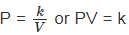Boyle's law can be verified by any one of the following three ways graphically.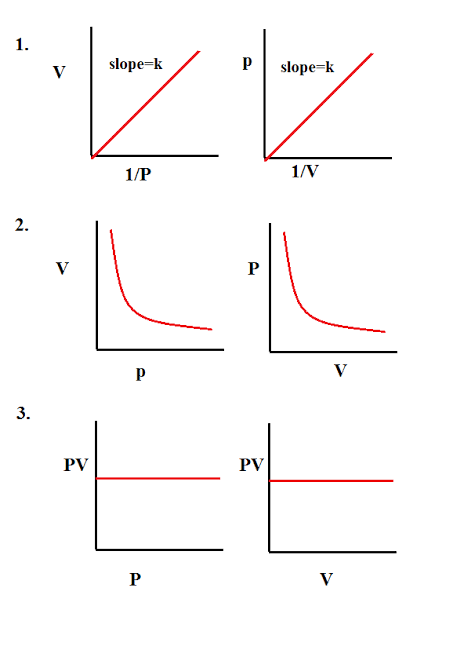Alternatively, Boyle's law can also be stated as follows :

"Temperature remaining constant , the product of pressure and volume of a given mass of a gas is constant".

The value of the constant depends upon the amount of a gas and the temperature.

Mathematically, it can be written as,

P1V1 = P2V2 = P3V3 = ..............

Location of straight line and curve changes with temperature in the isotherm shown in the following figure.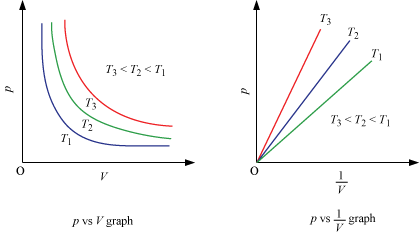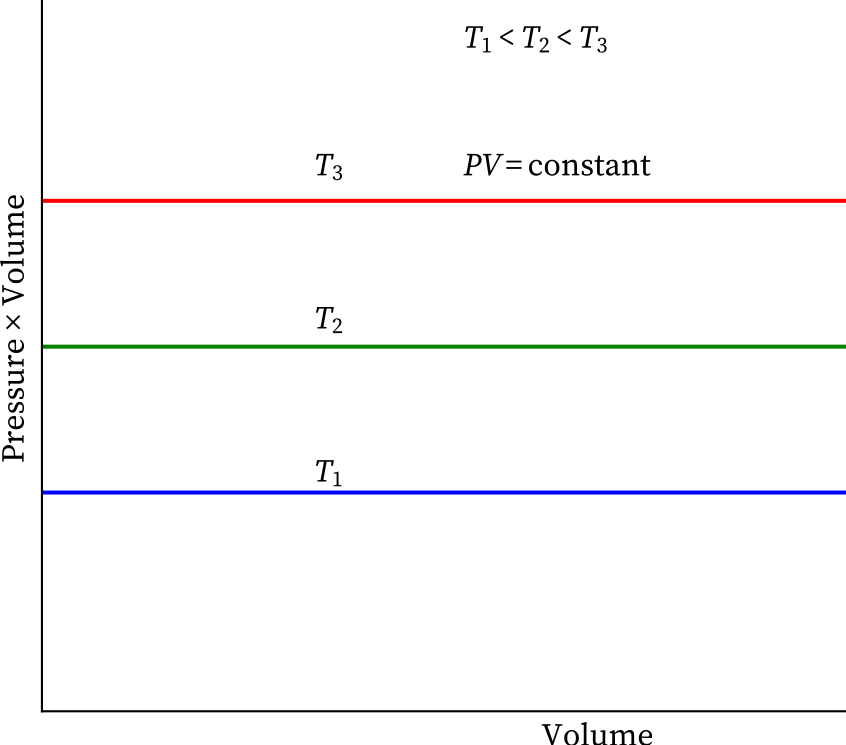According to Boyle's law, PV = Constant at constant temperature

∴ log P log V = constant

log P = -log V = constant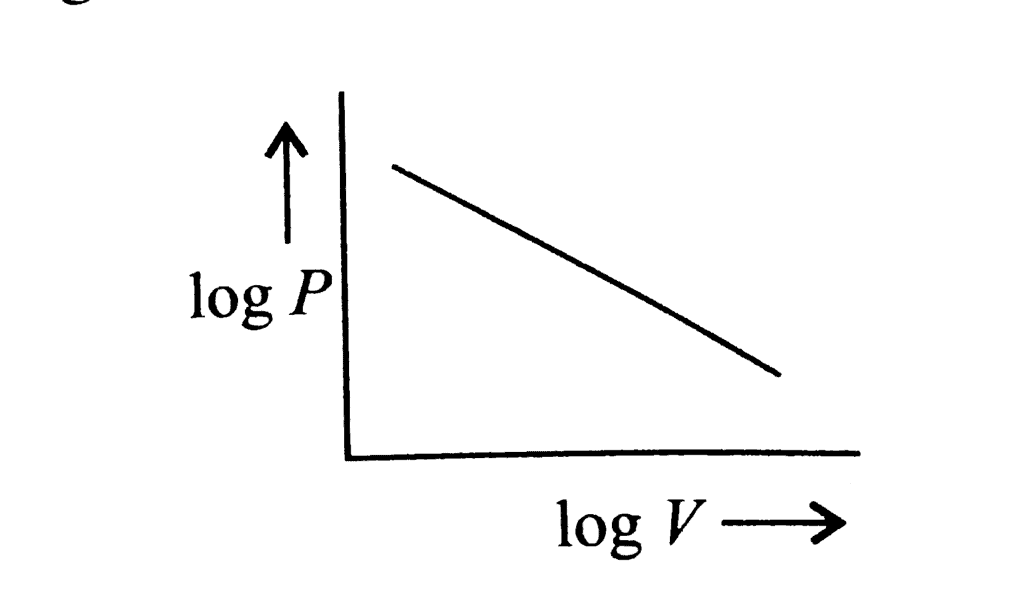Illustration 1: A gas is present at a pressure of 2 atm. What should be the increase in pressure so that the volume of the gas can be decreased to 1/4th of the initial value if the temperature is maintained constant.

Solution: PV = constant for a given mass of gas at constant pressure

⇒P1V1 = P2 V2

P1 = 2atm

V= V/4

P2 =?

Now,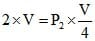⇒ P2 = 8 atm

∴ Pressure should be increased from 2 to 8 atm

∴ total increase = 8 - 2 = 6 atm.

Illustration 2: A sample of gas occupies 10 litre under a pressure of 1 atmosphere. What will be its volume if the pressure is increased to 2 atmospheres? Assume that the temperature of the gas sample does not change.

Solution: Mass and temperature is constant hence we can use Boyle's law

P1V1 = P2V2

Given P1 = 1 atm, V1 = 10 litre, P2 = 2 atm, V2 = ?

1 × 10 = 2 × V2 ⇒ V2 = 5 litre

Charles' Law

It relates the volume and temperature of a given mass of a gas at constant pressure.

For each degree change of temperature, the volume of a sample of a gas changes by the fraction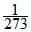of its volume at 0 °C.

Let the volume of a given amount of gas be V0 at 0°C. The temperature is increased by t°C and the new volume becomes Vt

Thus,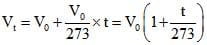or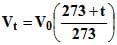A new temperature scale was introduced known as Kelvin scale or absolute scale (named after the British physicist and mathematician Lord Kelvin). The lower limit of the scale is called absolute zero which corresponds to - 273°C.

At absolute zero or - 273 °C, all molecular motions would stop and the volume of the gas would become zero. The gas would become a liquid or solid. Thus, absolute zero is that temperature at which no substance exists in the gaseous state. The temperature in absolute scale is always obtained by adding 273 to the temperature expressed in °C.

K = (t°C 273)

This new temperature scale may be used for deducing Charles' law.

By substituting T for 273 t and T0 for 273 in Eq. (i).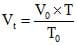or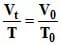or V/T = constant, if pressure is kept constant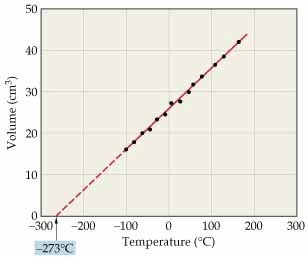(This graph is called isobar)

Alternatively, Charles' law can be stated as follows:

"The volume of a given amount of a gas at constant pressure varies directly as its absolute temperature".

V ∝ T (if pressure is kept constant)

Illustration 3: 1 mole of an ideal gas at constant atmospheric pressure is heated to increase its volume by 50% of initial volume. The change in temperature made was 300 K to T K. Then calculate final temperature.

Solution: At constant pressure for a given amount of gas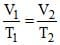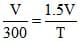(V1 = V, T1= 300 K, V2 = 1.5V)

T = 450 K

Pressure-Temperature Law (Gay Lussac's Law)

It relates the pressure and absolute temperature of a given mass of a gas at constant volume.

Volumes remaining constant, the pressure of a given mass of a gas increases or decreases byof its pressure at 0°C per degree change of temperature.

Pt = P0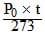or Pt = P0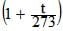or Pt = P0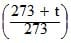= P0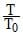or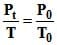or (if volume and number of moles are kept constant)

At constant volume, the pressure of a given amount of a gas is directly proportional to its absolute temperature.

Illustration 4: Pressure of gas contained in a closed vessel is increased by 0.4%, when heated by 1ºC. Calculate its final temperature. Assume ideal nature.

Solution: Since no information is given about volume hence we can assume that volume is constant and use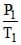=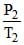given P1 = P, T1 = T, P2 = P = 1.004 P, T2 = T 1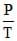=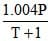⇒ T = 250 K

For Solid, Liquid and Gas

1 mole of any substance contains Avogadro's number (NA) of molecules/atoms/particles etc.

NA = 6.023 × 1023

For Gases

In 1812, Amadeo Avogadro stated that samples of different gases which contain the same number of molecules (any complexity, size, shape) occupy the same volume at the same temperature and pressure. It follows from Avogadro's hypothesis that V ∝ n (T and P are constant).

V ∝ n (T, P constant) ⇒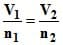STP: 273.15 K 1 atm

SATP: 298.15 K 1 bar

The document The Gas Laws - Notes | Study Chemistry Class 11 - NEET is a part of the NEET Course Chemistry Class 11.
All you need of NEET at this link: NEET

## Chemistry Class 11

204 videos|331 docs|229 tests

## Chemistry Class 11

204 videos|331 docs|229 tests

### How to Prepare for NEET

Read our guide to prepare for NEET which is created by Toppers & the best Teachers

Track your progress, build streaks, highlight & save important lessons and more!

,

,

,

,

,

,

,

,

,

,

,

,

,

,

,

,

,

,

,

,

,

;